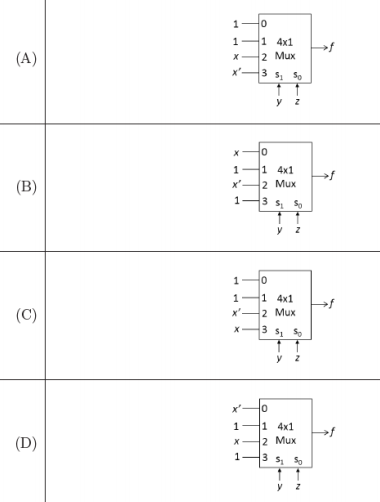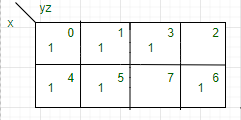Skip to content
Related Articles
GATE | GATE CS 2021 | Set 2 | Question 15
• Last Updated : 23 May, 2021

Which one of the following circuits implements the Boolean function given below?

`f(x,y,z) = m0 + m1 + m3 + m4 + m5 + m6 `

where mi is the ith minterm.(A) A
(B) B
(C) C
(D) D

Answer: (A)

Explanation:Above is the k-map of the given boolean function

Now represent min terms in binary form

`= 000, 001, 011, 100, 101, 110`

Sort by last 2 digits because select lines are 2 only.

`= 000, 100, 001, 101, 110, 011`

= Now on substituting the value of x and x’

We will get I0 = 1, I1 = 1, I2 = x and I3 = x’ (Correct Option A)

Attention reader! Don’t stop learning now. Learn all GATE CS concepts with Free Live Classes on our youtube channel.

My Personal Notes arrow_drop_up